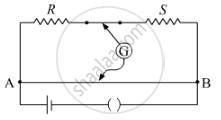# In a Meter Bridge Shown in the Figure, the Balance Point is Found to Be 40 cm from End A. If a Resistance of 10 Ω is Connected in Series with R, Balance Point is Obtained 60 cm from A. Calculate the Values of R and S. - Physics

In a meter bridge shown in the figure, the balance point is found to be 40 cm from end A. If a resistance of 10 Ω is connected in series with R, balance point is obtained 60 cm from A. Calculate the values of R and S.#### Solution

When balance point is at 40 cm, we have

RS=40/(100−40)=40/60

=>R/S=2/3

=>3R=2S...........(i)

When a resistance of 10 Ω is added in series with R,Then, equivalent reistance at R is R=R+10Now, balance point is at 60 cm.So, we have

(R+10)/S=60/(100−60)=60/40

(R+10)/S=3/2

2R+20=3S

2R3S=20   .....(ii)

Solving (i) and (ii), we get

S=12 Ω

R=8 Ω

Concept: Metre Bridge
Is there an error in this question or solution?
2014-2015 (March) Patna Set 2

Share